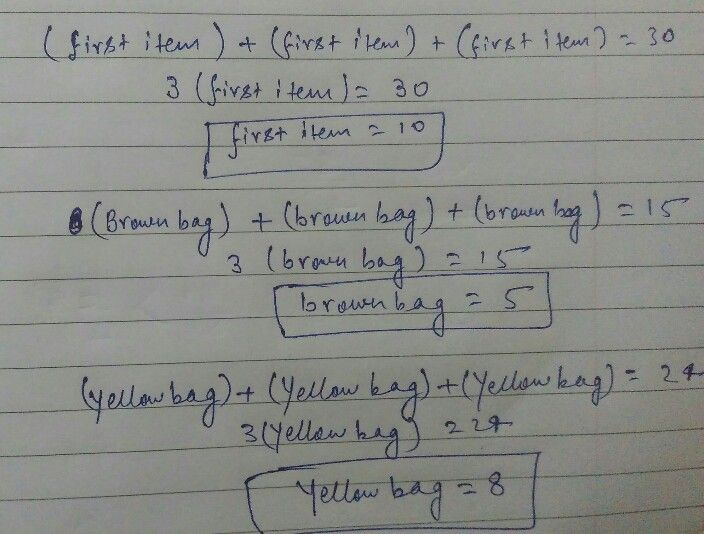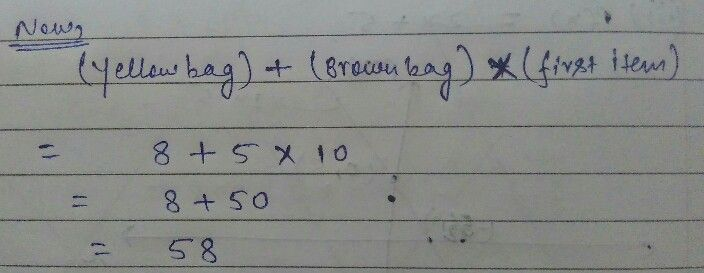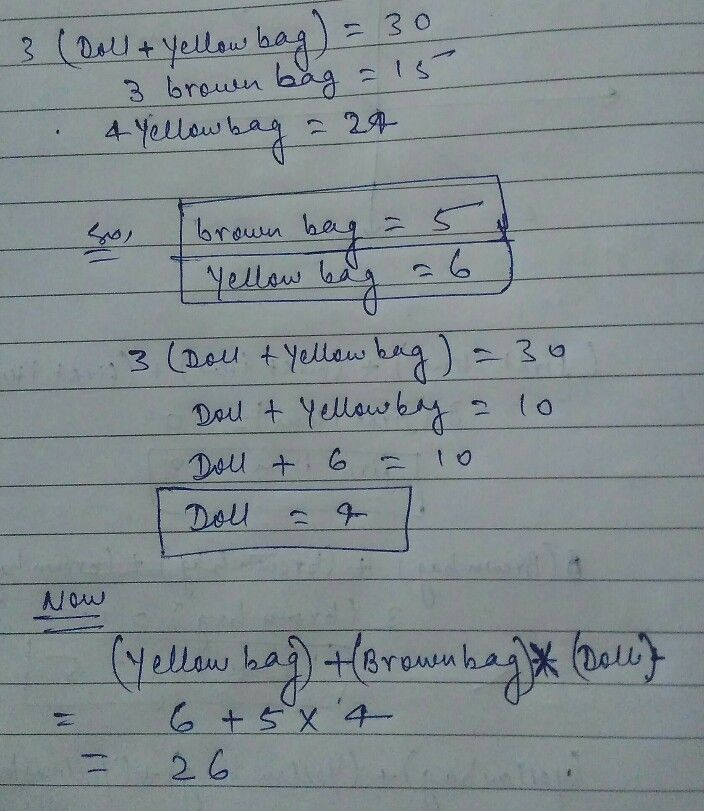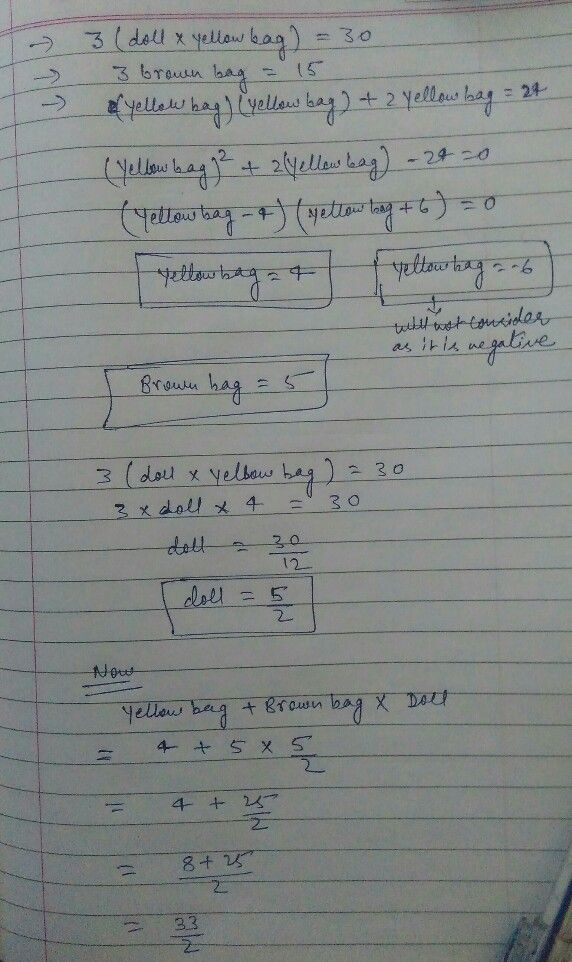Symbol
Problem$+$ $2^{G2}$ $+$ $\bar{2} ^{2}=30$ $\square$ $+$ $\square$ $5x$ $2$ $=15$ $4$ $4$ $4t$ $=24$ $4t$ $8$ $2$ $=3$
Other
SolutionQanda teacher - Amit Arunplease let me know if you have any queryStudent
do u see the yellow thing in the girl and it disappear in third
and the yellow bag the first one is doubleQanda teacher - Amit Arun
I think that will be typo error if you want me to consider that
we can consider yellow bag as double but for the yellow thing in the doll hand can't be considered any value
but if you want to get solve like that will solve thisStudent
okay let get answer with double bag and then try to get in dollQanda teacher - Amit Arun
okay please , will solve with everything nowthis is including extra yellow bag and also including doll having yellow bagStudent
are u sure thatQanda teacher - Amit Arun
if you include then this will be answerStudent
you made boy and girl with 4 are u sureQanda teacher - Amit Arun
boy and girl is considered as single doll and the doll is having yellow bag
so (doll + yellow bag)*3 = 30Student
okay thank you very much you helped me so much but it really diffcult
if you have any idea more tell meQanda teacher - Amit Arun
one more way to solve it
like doll is having yellow bagStudent
okay tell them and we try to choose the right oneQanda teacher - Amit Arun
so you can multiply doll and yellow bag .
wait will solve and send you
but again the answer will be differentStudent
no propelem i will take 2 from the answerQanda teacher - Amit Arunyou can check this alsoStudent
okay thank you very much with helping i will take this one and the other
i hope to be right
thank youQanda teacher - Amit Arun
welcome